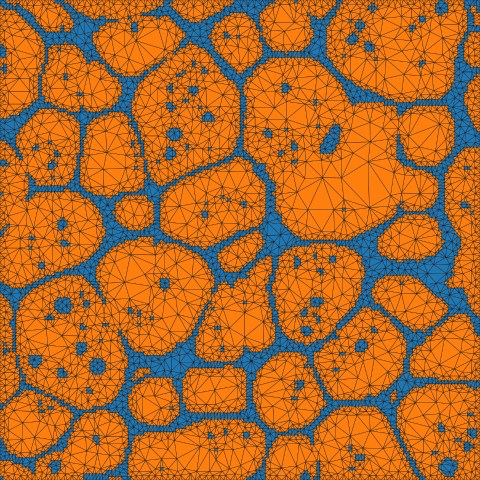# Microstructure from Image¶

Note

The open source and freely available software package OOF is better equiped to create unstructured meshes from images.

https://www.ctcms.nist.gov/oof/

## Python Script¶

The basename for this file is `from_image.py`. The file can be run using this command:

```microstructpy --demo=from_image.py
```

The full text of the script is:

```import os
import shutil

import numpy as np
from matplotlib import image as mpim
from matplotlib import pyplot as plt

import microstructpy as msp

# Read in image
image_basename = 'aluminum_micro.png'
image_path = os.path.dirname(__file__)
image_filename = os.path.join(image_path, image_basename)
im_brightness = image[:, :, 0]

# Bin the pixels
br_bins = [0.00, 0.50, 1.00]

bin_nums = np.zeros_like(im_brightness, dtype='int')
for i in range(len(br_bins) - 1):
lb = br_bins[i]
ub = br_bins[i + 1]
mask = np.logical_and(im_brightness >= lb, im_brightness <= ub)

# Define the phases
phases = [{'color': c, 'material_type': 'amorphous'} for c in ('C0', 'C1')]

# Create the polygon mesh
m, n = bin_nums.shape
x = np.arange(n + 1).astype('float')
y = m + 1 - np.arange(m + 1).astype('float')
xx, yy = np.meshgrid(x, y)
pts = np.array([xx.flatten(), yy.flatten()]).T
kps = np.arange(len(pts)).reshape(xx.shape)

n_facets = 2 * (m + m * n + n)
n_regions = m * n
facets = np.full((n_facets, 2), -1)
regions = np.full((n_regions, 4), 0)
region_phases = np.full(n_regions, 0)

facet_top = np.full((m, n), -1, dtype='int')
facet_bottom = np.full((m, n), -1, dtype='int')
facet_left = np.full((m, n), -1, dtype='int')
facet_right = np.full((m, n), -1, dtype='int')

k_facets = 0
k_regions = 0
for i in range(m):
for j in range(n):
kp_top_left = kps[i, j]
kp_bottom_left = kps[i + 1, j]
kp_top_right = kps[i, j + 1]
kp_bottom_right = kps[i + 1, j + 1]

# left facet
if facet_left[i, j] < 0:
fnum_left = k_facets
facets[fnum_left] = (kp_top_left, kp_bottom_left)
k_facets += 1

if j > 0:
facet_right[i, j - 1] = fnum_left
else:
fnum_left = facet_left[i, j]

# right facet
if facet_right[i, j] < 0:
fnum_right = k_facets
facets[fnum_right] = (kp_top_right, kp_bottom_right)
k_facets += 1

if j + 1 < n:
facet_left[i, j + 1] = fnum_right
else:
fnum_right = facet_right[i, j]

# top facet
if facet_top[i, j] < 0:
fnum_top = k_facets
facets[fnum_top] = (kp_top_left, kp_top_right)
k_facets += 1

if i > 0:
facet_bottom[i - 1, j] = fnum_top
else:
fnum_top = facet_top[i, j]

# bottom facet
if facet_bottom[i, j] < 0:
fnum_bottom = k_facets
facets[fnum_bottom] = (kp_bottom_left, kp_bottom_right)
k_facets += 1

if i + 1 < m:
facet_top[i + 1, j] = fnum_bottom
else:
fnum_bottom = facet_bottom[i, j]

# region
region = (fnum_top, fnum_left, fnum_bottom, fnum_right)
regions[k_regions] = region
region_phases[k_regions] = bin_nums[i, j]
k_regions += 1

pmesh = msp.meshing.PolyMesh(pts, facets, regions,
seed_numbers=range(n_regions),
phase_numbers=region_phases)

# Create the triangle mesh
tmesh = msp.meshing.TriMesh.from_polymesh(pmesh, phases=phases, min_angle=20)

# Plot triangle mesh
fig = plt.figure()
ax = plt.Axes(fig, [0., 0., 1., 1.])
ax.set_axis_off()
ax.get_xaxis().set_visible(False)
ax.get_yaxis().set_visible(False)

fcs = [phases[region_phases[r]]['color'] for r in tmesh.element_attributes]
tmesh.plot(facecolors=fcs, edgecolors='k', lw=0.2)

plt.axis('square')
plt.xlim(x.min(), x.max())
plt.ylim(y.min(), y.max())
plt.axis('off')

# Save plot and copy input file
plot_basename = 'from_image/trimesh.png'
file_dir = os.path.dirname(os.path.realpath(__file__))
filename = os.path.join(file_dir, plot_basename)
dirs = os.path.dirname(filename)
if not os.path.exists(dirs):
os.makedirs(dirs)

shutil.copy(image_filename, dirs)
```

The first section of the script reads the image using matplotlib. The brightness of the image is taken as the red channel, since the RGB values are equal. That image is shown in Fig. 25.

## Bin Pixels¶

The pixel values are binned based on whether the brightness is above or below 0.5.

## Phases¶

The two phases are considered amorphous, to prevent pixilation in the triangle mesh.

## Create the Polygon Mesh¶

The polygon mesh is a reproduction of the pixel grid in the image. The facets are edges between pixels, and the polygons are all squares.

## Create the Triangle Mesh¶

The triangle mesh is created from the polygon mesh and uses the amorphous specifications for the phases. The minimum interior angle of the triangles is set to 20 degrees to control the aspect ratio of triangles.

## Plot Triangle Mesh¶

The axes of the plot are switched off, then the triangle mesh is plotted. The color of each triangle is set by the phase.

## Save Plot and Copy Input File¶

The final plot is saved to a file, then the input image is copied to the same directory for comparison. The output PNG file of this script is shown in Fig. 26.Fig. 26 Triangular mesh of aluminum microstructure.¶